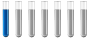HelpAuthor Message

NewbieJoined: 19 Mar 2018
Posts: 1Help

Error: equation K(MnO4)+H2O2+H2SO4=K2(SO4)+Mn(SO4)+O2+H2O can be balanced in an infinite number of ways: this is a combination of two different reactions..... Why ? K(MnO4)+H2O2+H2SO4=K2(SO4)+Mn(SO4)+O2+H2O
I need a better explanation. PleaseDistinguished MemberJoined: 27 Sep 2017
Posts: 287
Location: Berlin, GermanyIt is a redox reaction. MnO4 - + 8 H+ + 5 e- => Mn 2+ + 4 H2O Reduction H2O2 => 2 H+ + O2 + 2 e - is the Oxidation. Potassium and Sulfate are spectator ions and don't change. From this two equations you should develop the main reaction.Display posts from previous: All Posts1 Day7 Days2 Weeks1 Month3 Months6 Months1 Year Oldest FirstNewest FirstPage 1 of 1